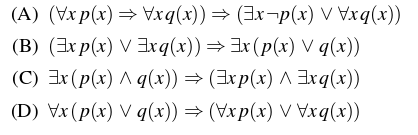# GATE | GATE-CS-2016 (Set 2) | Question 37

Which one of the following well-formed formulae in predicate calculus is NOT valid?(A) A
(B) B
(C) C
(D) D

Explanation:

```Suppose if there are two statements P and Q,
P=>Q = ~PvQ i.e.
The only situation where implication fails is (=>) when P is true and Q is false.
i.e. A truth statement can't imply a false statement.
So, for these type of questions it will be better to take option and
check for some arbitrary condition
By looking options, we are pretty sure that A,B are correct
Suppose X is any number and statement is P(x) = X is a prime number
Q(x) = X is a non-prime number
If we look at option D,
Before Implication :- For all x, x is either prime or non-prime which is true
After Implication  :-   For all x, x is prime or for all x, x is non-prime which is
obviously false i.e. here, truth statement implies a false statement which is not valid.

If we carefully look at option C,
There exists a number x, which is both prime and non-prime which is false
and a false statement can imply either true or false. So option (C) is correct